Inverted nine

In the hotel,, Inverted nine" each hotel room number is divisible by 6. How many rooms we can count with three-digit number registered by digits 1,8,7,4,9?

Result

n =  18

Solution:Leave us a comment of example and its solution (i.e. if it is still somewhat unclear...):Be the first to comment!Next similar examples:

1. PupilsThere are 27 pupils in the classroom. They can swim 21 and ski nine pupils. Neither swim nor ski three pupils. How many pupils can swim and ski?
2. Chocolate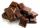Leslie bought 8 same chocolates for 16 Eur. How many euros will he pay for 25 chocolates?
3. Each with each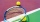Five pupils from 3A class played table tennis. How many matches did they play with each other?
4. Temperature change 2The outside temperature changed -14F over 4 hours. If the temperature changed the same amount each hour, what was the change in temperature each hour?On the menu are 12 kinds of meal. How many ways can we choose four different meals into the daily menu?
6. Number of songs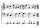Write an expression for the number of songs they need for this show. Evan and Peter have a radio show that has 2 parts. They need 4 fewer than 11 songs in the first part. In the second part, they need 5 fewer than 3 times the number of songs in the first p
7. DigitsHow many odd four-digit numbers can we create from digits: 0, 3,5,6,7?
8. Bookshelf and books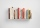How many can we place 7 books in a bookshelf?
9. Expression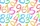If A=2 B=3 evaluate expression A(B+A) and multiply it by A
10. Brick weightThe brick weighs 2 kg and a half bricks. How much does one brick weigh?
11. Football team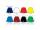Football team has in store black, purple and orange shirts, blue and white shorts and striped and gray socks. How many different outfits players may start?
12. Speed of carIn 2 hours 40 mins, a car travels 100km. At what speed is the car traveling?
13. One threeWe throw two dice. What is the probability that max one three falls?
14. Photo frameA square shape photo with a side length of 20 cm is framed by a 4 cm wide bar. Find the external frame size of this photo (the frame is from all sides)In about 12 hours in North Dakota the temperature rose from -33 degrees farenheit to 50 degrees farenheit. By how much did the temperature change?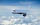The students vice adventure had a 2,367 km flight. If they travel time was 2 hours and 56 minutes, what was their average speed in kilometres per hour?Starting temperature is 21°C, the highest temperature is 32°C. What is the change in temperature?